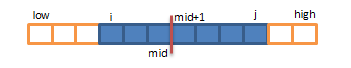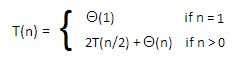# Solution of Maximum Sub Array Problem like how to find maximum subarray in an array

 Category: Data Structure And Algorithms Tags:Maxim Sub Array Problem Code Files

## Introduction

Problem Statement: Sometimes we are given an array of n integers and array can contain positive and negative both type of values. It can be asked to find the continuous sub-array which has maximum sum of all elements than any other possible sub-array drawn from the given array. For an example we have an array like: A = [1,-2,3,4,-6] and if we see the max sub-array will be Amax=[3,4] which sum is 3+4=7. if we take any other sub-array like [1,-2,3] (Sum=2) or [-2,3,4,-6] (Sum=-1). So our solution is Amax= [3,4].

The next question may come in your mind is, why would it be required to find maximum sub-array of given array? So answer is, yeah it is useful to solve some interesting problems like:

Suppose a company called XYZ wants to know in between which years it had maximum profit, and the data is given:

 Year 1 2 3 4 5 6 Profit(M\$) -2 -1 3 -2 6 -3

From above data we try to find out the maximum sub array that is [3,-2,6] in years between 3-5. So we can say the company XYZ's maximum profit was in years between 3 to 5. And here we can see the requirement of finding maximum sub-array.

## Solution by Divide and Conquer:

Lets suppose we are having an array of n elements A[1,2,3,4.....n] so mid is n/2 or we re-write it as A[low ...mid ...high], so where we might find the maximum sub-array? there may be 3 possible cases where max sub-array(let sub array is between index i and j) might be encountered:

1. It might be in [low, mid] so (low ≤ i ≤ j ≤ mid)
2. It might be in [mid+1, high] so (mid+1 ≤ i ≤ j ≤ high)
3. Or it might be in both at a time means it might be crossing the mid point, so
(low ≤ i ≤ mid < j ≤ high)

*Because we are making sub problems by dividing the problem so we can solve the problem easily in recursive manner only we need to find maximum sub array which crosses the mid and then we will compare all these three and return which is maximum of them.## Implementation:

To Implement we will create two methods called FindMaxCrossingSubArray which will find the possible sub array with maximum sum and crossing the mid, the other one FindMaxSubArray will break an array from mid. We will create a property class to store the indexes and sum of maximum array.

```public class MaxArrayResult
{
public int IndexMin { get; set; }
public int IndexMax { get; set; }
public int Sum { get; set; }
}
```

The above class we will use to store details about maximum sub array. Now we will create a class called MaxSubArray which will contain the array we have to sort and required methods for sorting:

```public class MaxSubArray
{
int[] A;
public MaxSubArray(int[] array)
{
A = array;
}
public MaxArrayResult FindMaxSubArray(int low, int high){ ... }
public MaxArrayResult FindMaxCrossingSubArray(int low, int high, int mid) { ... }
}
```

Below we are giving implementation of FindMaxCrossingSubArray method

```public MaxArrayResult FindMaxCrossingSubArray(int low, int high, int mid)
{
MaxArrayResult result = new MaxArrayResult();
int sum = A[mid];       // First half of the array
int leftSum = A[mid]; // will track sum till current node
int leftMax = mid;   // will track the node till it finds maximum sum
for (int i = mid - 1; i >= low; i--) // going from mid to zero
{
sum = sum + A[i];
if (sum > leftSum)
{
leftSum = sum;
leftMax = i;
}
}
int rightSum = A[mid + 1];  //2nd half of the array
int rightMax = mid + 1;
sum = A[mid + 1];
for (int j = mid + 2; j <= high; j++) // going from mid+1 to last
{
sum = sum + A[j];
if (sum > rightSum)
{
rightSum = sum;
rightMax = j;
}
}

result.IndexMin = leftMax;    result.IndexMax = rightMax;    result.Sum = leftSum + rightSum; // Sum of left and right sub array
return result;
}
```

The above code shows how we find the sub-array which crosses the mid and having maximum sum, we have two loops which run from mid to both of the directions till respective ends to find maximum sub array. Now to find mid point and compare which is the maximum array, we have a method called FindMaxSubArray given below:

```public MaxArrayResult FindMaxSubArray(int low, int high)
{
if (low == high) // devides untill only one element is left
{
MaxArrayResult result = new MaxArrayResult();
result.IndexMin = low;
result.IndexMax = high;
result.Sum = A[low];
return result;
}

int mid = (low + high) / 2; // getting mid
/*next 2 steps are recursion*/
MaxArrayResult resultLeft = FindMaxSubArray(low, mid); //generating result for left array
MaxArrayResult resultRight = FindMaxSubArray(mid + 1, high); //generating results for right
/*calling above method*/
MaxArrayResult resultMid = FindMaxCrossingSubArray(low, high, mid); // for middle one
/*below steps compare results and return largest sum*/
if (resultLeft.Sum >= resultRight.Sum && resultLeft.Sum >= resultMid.Sum)
return resultLeft;
else if (resultRight.Sum >= resultLeft.Sum && resultRight.Sum >= resultMid.Sum)
return resultRight;
else
return resultMid;
}
```

The above code exactly divides the array in two sub arrays and finds the maximum sub arrays of all the scenarios stated early in the article and based on the results it returns the sub-array which has greatest sum

```static void Main(string[] args)
{
int[] A = new int[] { -2, -1, 3, -2, 6, -3 };
MaxSubArray mArray = new MaxSubArray(A);
MaxArrayResult r = mArray.FindMaxSubArray(0, A.Length - 1);
Console.WriteLine("max sub array low index = " + r.IndexMin);
Console.WriteLine("max sub array high index = " + r.IndexMax);
Console.WriteLine("Sum of Sub Array = " + r.Sum);
Console.ReadLine();
}
```

Output:

max sub array low index = 2

max sub array high index = 4

Sum of Sub Array = 7

Here it printed low index is 2 which is actually year-3(array's starting index is 0 so) and high index is 4 so it will be year-5 so in year 3 to 5 the company XYZ had maximum profit which is 7M\$.

## Analysis

Recurrence will be:Because the method FindMaxCrossingSubArray will run in linear time Θ(n) and the method FindMaxSubArray will be dividing the array in 2 sub arrays. So

T(n) = 2T(n/2) + cn          ...eq1

if replace n by n/2

=> T(n/2) = 2T(n/4) + c(n/2)

substituting value of T(n/2) in eq1

=> T(n) = 2[ 2T(n/4) + c(n/2) ] + cn

=> T(n) = 22T(n/22) + 2cn

if we go so far

=> T(n) = 2kT(n/2k) + k.cn

if at some value 2k = n then k = log2 n, so

=> T(n) = n Θ(1) + log(n).cn

=> T(n) = n + n.log n

So simply we can say the complexity of finding maximum sub array is Θ(n log n).

 Like0 People
Last modified on 29 October 2018Nikhil Joshi Ceo & Founder at Dotnetlovers Atricles: 132 Questions: 9 Given Best Solutions: 9 *

## Comments:

No Comments Yet

You are not loggedin, please login or signup to add comments:

## Existing User

Login via:## New Userx